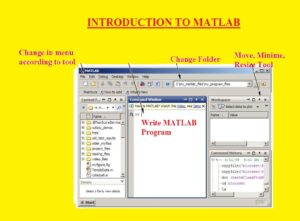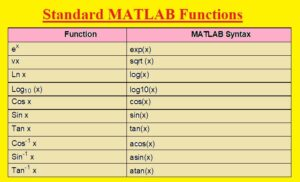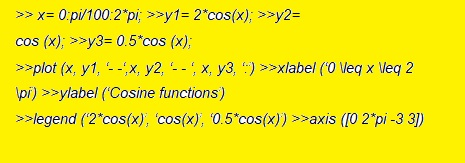Hello, readers welcome to the new post. In this post, we will discuss INTRODUCTION TO MATLAB. MATLAB is software that is used to perform data manipulation calculations visualization math and programming.  This software has features to handle basic and high-level operations in the simple and easiest way. It is mostly used for the solution of differential equations and optimization of different operations.

Through the use of this software, we can solve larger systems of equations effectively and so it is used as the finest option to solve differential equations. We can also get data visualization and symbolic features from this software. Through this software, we can solve easy equations effectively but it has features to resolve high-level operation that has complications. In this post, we will cover different parameters for MATLAB and related problems. So let’s get started INTRODUCTION TO MATLAB

### Common Uses of MATLAB

• MATLAB is the finest option to solve linear algebra and matrix computation. It is a very famous solution for industrial solution engineers used to solve different research-based projects and math problems.
• With that this software can also be used for automatic control solutions, statistics, and digital signal processing.
• The common uses of this software for industries and scientific-based projects are listed below
• Signal Processing
• Communication System
• Control Systems
• Fuzzy Logic
• Wavelets
• Generic Algorithms
• Optimization
• Neural Networks

#### How to Use MATLAB

• First of all, you must have MATLAB installed on your computer. Then press on the MATLAB icon to start it.
• When you press on the Icon MATLAB screen will be shown. In the below figure, you can see the interface. The MATLAB desktop is also called IDE or Integrated Development Environment.
• In the below screen, there is a start button exist in the lower left-side corner that will be shown the MTALB tools, shortcuts, and documentation### How to Use MATLAB as Calculator

• To use MATLAB as a calculator we solve simple equations in the software. Let solve an expression
• we have 2+5*2
• to solve this equation write in prompt command(>>) as below
• 2+5*2 ans=12

### MATLAB Mathematical Functions

• In the below table, you can see the standard MATLAB FunctionsLet’s solve an example with these functions

y= e-a sin(x) + 10√y

for a=5, x=2, and y=8 is computed by

>a=5; x=2; y=8;

>y=exp (-a)*sin(x) +10*sqrt(y)

y=

28.2904

• Let’s solve another example

To find the value of sin (pi/4) and e10

>sin(pi/4)

ans=

0.7071

>>exp(10)

ans=

2.2026e+004

### MATLAB Variables, Expression, and Statements

• Variable is one word having no space and numbers of characters up to 31. These variables are sensitive to the case and their names start with a letter. There is no use of punctuation characters
• MATLAB statements are written as

>>Variables= Expression

>>Expression

• Expressions consist of function operators and variable names. When the evaluation is done value is given to the variable and shown. If the variable name and =sign are not added the answer of the variable is automatically generated and the result will be assigned to ans

### Precedence Rules

• Expressions are solved from left to right through the use of exponential operation with a high value of precedence, after that multiplication and division with equal precedence then addition at last subtraction with equal precedence.
• There is the use of parentheses to change this sequence for which case these rules of precedence are functioned in every set of parentheses initiating with the inner set and preceding to forward.
• The value that was added recently given to the variable that was used is given here. Let’s suppose if we write x at the prompt that output will be

>>X

X =

7

• The numerical value can be seen in another format that is mentioned below

e= 1/3

e = 0.3333

• format long (long decimal format)

e

e = 0.33333333333333

format short e (short exponential format)

e

e =

3.3333 e-01

>>format long e (long exponential format) e =

3.33333333333333 e-04

>format ( default format)

>e

e =

0.333

### Matrices in MATLAB

• There are different ways to enter the matrices in MATLAB. Which are listed below
• Write Explicit elements lists
• Through the use of built-in functions produce the matrices
• Make matrices through the use of functions and then fave in files
• The operation of MATLAB is based on a single type of object like a rectangular numerical matrix with complex entries.
• All variable denotes the matrices. If we have to store matrix 1 2 3 in variable x through the use of MATLAB we have to write the following code• For row, separation semicolons are used and for elements, separation space or commas are used. So above shown matrix can be stored through the use of the below command

>X = [1,2,3;4,5,6;7,8,9];

• For this purpose, the statement can also use

X = [ 1 2 3 4 5 6 7 8 9 ]

• The existence of a
• semicolons at the command end suppress the results
• Here transpose of matrix b is taken and stored in y through the below command

>>Y = X’

Y =

1 4 7

2 5 8

3 6 9

• Matrices operations performed in the MATLAB are seen here

### Matrix Operations In MATLAB

>>X=[1 2 3 4; 5 6 7 8]

X=

1 2 3 4

5 6 7 8

X(:,:)

Ans=

1 2 3 4

5 6 7 8

X(1,2)

Ans=

2

>X(1,:)

Ans=

1 2 3 4

X(:,[1,3])

Ans=

1 3

5 7

>>X=(:,end)

Ans=

3

7

• You can assigned to new matrix to selected part of matrix

>>y=X(:,3:end)

y=

3 4

7 8

• To choose the elements of the main diagonal

>>Diag(Y)

Ans=

3

8

Changing and deleting matrix elements

>>X= 1:5

1 2 3 4 5

>>X(2)=6

X=

1 6 3 4 5

>>X([1 3])=0

X=

0 6 0 4 5

### MATLAB Matrix Manipulating

>>X=[1 2 4: 5 7 8]

X=

1 3 4

5 7 8

To take transpose

>>X’

Ans=

1 5

3 7

4 8

>fliplr (X)

Ans=

4 3 1

8 7 5

>flipud(X)

Ans=

5 7 9

1 3 4

### Matrix Operation

>>X= [1 3 4; 5 7 8]

X=

1 3 4

5 7 8

> 3+X

Ans=

3 6 7

8 10 11

### Element-by-element operations vs. Matrix Operations

• Certain types of measure taken for Matrix multiplication and has differenece from basic element to element multiplication.
• So we have certain froup of operators which differentiate the matrix operation from elementy by element opeation. For perform the matrix operation use * for multiplication, / for division , ^ for exponentiation.
• While for side by side opeation we uses .* for multiplcation, ./ for division and .^ for exponentation.

>>X=[2 3 4; 5 6 7; 2 1 0];

>>Y=[1 2 3; 5 6 2; 0 0 5];

>X*Y

Ans=

Ans=

17 22 32

40 52 59

7 10 8

>>X*Y

2 6 12

25 42 12

0 0 0

### Plotting in MATLAB

• MATLAB software comes in different graphic tools.  Through the use of less commands, we can plot the given data into a graphical representation
• Graphical representation of data is very simple and easy in MATLAB.
• Here we will discuss the data plotting in the MATLAB
• For plotting the graph command of Matlab is “plot(x,y)”. Let’s suppose we have two vectors  x= (1; 2; 3; 4; 5; 6) and y= (3; -1; 2; 4; 5; 1)

>> x= [1 2 3 4 5 6];

>> y= [3 -1 2 4 5 1];>>plot(x,y)

The result is given here• Now we solve this below expression

>>X=0

Now try the following:

>>x = 0: pi/100: 2*pi; >>y= sin (x);

plot (x,y)

### Axis Labels and Adding Titles

• Through the use of Matlab, we can add titles and labels axis. For example for the previous example we add X- and Y-axis labels

>> label (‘x = 0:2\pi’); >>ylabel (‘sine of x’);

>>title (‘Plot of the Sine Function’)

### MATLAB multiple Data Set in a Single Plot

• Through the use of a single-cell plot many (x,y) pairs of expressions are created. For example, this expression draws three plots for functions  x: y1=2 cos(x), y2=cos(x), and y3=0.5*cos (x) in the interval 0 ≤ x ≤ 2∏• The output of our code is shown below### Introduction to MATLAB Programming

• For the creation of file in MATLAB press on New in the file menu in the menu bar then you will see the new file tab
• When you write the code and have to save the file the called m-file will be saved in bin folder of MATLAB by default function
• These files is known as M-Files since they have ”.m” in the title. There are two types of M-files Script files and Function files

Here sample M-File exist

x=[1,2,3;0,1,1;1,2,3]

y=x’;

z= x+y

a=inv(z)

### What is the Function Files

• For extension of Matlab functions files used. We can make new functions according to our issue that will have a similar status like other MATLAB functions.
• Variables in the function file are through default local. Though we can make a variable globel according to requirements
• Let solve example
• Function X=prod(c,d)

X= c*d

• Resolve this file having filename prod.m after that write MATLAB prompt

>>prod(2,4) ans=

8

• To get the input from users syntax rule is applied

>>variable name = input (‘variable’);

• Let us have another example

>>a=input (‘ Enter a number ‘);

• And for the command prompt, if we function this code it will be shown like:>> Enter a number
• if we write 1 at the cursor will have a result like x=1

### MATLAB Control FLOW

• MATLAB has below control flow structures
• If- Else –End
• For loops
•  While loops
• Switch-Case constructions

### What is Script Files

• The script file has a series of normal MATLAB statements. if the file has a name like rkg.m then MATLAB command>>rkg will result in statements in the file to be operated.
• The script file has global variables and will vary the value of variables of the same title in conditions of the current MATLAB section
• Larger matrix solutions are done by script files

### What is Load Function MATLAB

• The load function of MATLAB reads binary files consisting of matrices produced by previous MATLAB sessions or reads text files comprising numeric data.
• A text file must be configured in the rectangular table having numbers, parted by blanks having one row in one line and an equal number of elements in every row.

That is all about the MATLAB Software all details has explained if you have any queries ask here